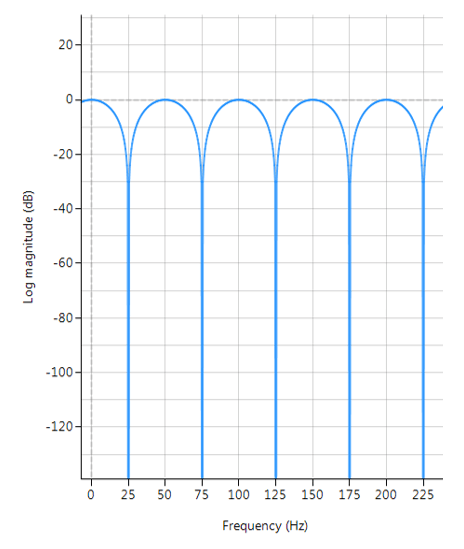# FIR Comb Filter

Comb filters have found use as powerline (50/60Hz) harmonic cancellation filters in audio applications, and form the basis of so called CIC (cascaded integrator–comb) filters used for anti-aliasing in decimation (sample rate reduction), and anti-imaging in interpolation (sample rate increase) applications.

The frequency response of a comb filter consists of a series of regularly-spaced troughs, giving the appearance of a comb. As seen in the plot below, the spacing of each trough appears at either odd or even harmonics of the desired fundamental frequency.Frequency response of a typical FIR comb filter (odd harmonics cancellation):
$$f_s=500Hz$$,  $$f_c=25Hz$$, $$L=10$$ and $$\alpha=1$$

An FIR comb filter can be described by the following transfer function:

$$H(z)=1+\alpha z^{-L}$$
$$\Rightarrow Y(z)=X(z)\left[1+\alpha z^{-L}\right]$$

Clearly, the comb filter is simply a weighted delayed replica of itself, specifiied by $$L$$. Taking inverse z-transforms, we obtain the difference equation needed for implementation,

$$y(n)=x(n)+\alpha x(n-L)$$

where, $$\alpha$$ is used to set the Q (bandwidth) of the notch and may be either positive or negative depending on what type of frequency response is required. In order to elaborate on this, negative values of $$\alpha$$ have their first trough at DC and their second trough at the fundamental frequency. Clearly this type of comb filter can be used to remove any DC components from a measured waveform if so required. All subsequent troughs appear at even harmonics up to and including the Nyquist frequency.

Positive values of $$\alpha$$ on the other hand, only have troughs at the fundamental and odd harmonic frequencies, and as such cannot be used to remove any DC components.

## Application to powerline interference cancellation

The affectivity of the comb filter is dependent on the sampling frequency, $$f_s$$, as $$L$$ is limited to integer values only. Also, a relationship between $$f_s$$, as $$L$$ and will be dependent on the sign of $$\alpha$$. Thus, for the purposes of the mains cancellation application considered in this discussion, only positive values of will be considered, as we need only cancel odd harmonics.

A simple relationship for determining  $$L$$ can be summarized for positive values of $$\alpha$$ as follows:

$$L=ceil\left( \large{\frac{f_s}{2f_c}}\right)$$

where, $$f_c$$ is the desired centre point of the fundamental notch frequency. Based on this expression, we can re-calculate the sampling frequency, such that $$f_c$$ is a true multiple of $$f_s$$

$$f_{snew}=2f_c L$$

## Example

For the example considered herein, i.e. $$f_s=500Hz$$ and $$f_c=25Hz$$, we obtain $$L=10$$. However, if $$f_c=60Hz$$, we would need $$L=5$$, and a new sampling rate of $$600Hz$$ respectively, although it’s interesting to note that $$f_s=480Hz$$ for $$L=4$$ would also suffice.

## Implementation

An FIR comb filter may be implemented in ASN FilterScript as follows:

ClearH1;  // clear primary filter from cascade
interface L = {4,20,1,5}; // delay
interface alpha = {-1,1,0.01,1};

Main()
Num = {1,zeros(L-1),alpha}; // numerator coefficients
Den = {1};
Gain = 1/sum(abs(Num));0 replies